# 概述

• Web服务具有动态更改内容池的功能，从而使传统的协同过滤算法无法使用。
• 大多数具有实际意义的Web服务要求具有快速学习和进行计算

• 简单的线性不相交模型 disjoint LinUCB
• 混合相交的线性模型 hybrid LinUCB

# Disjoint LinUCB

$E[r_{t,a} | x_{t,a} ] = x^T_{t,a} \theta^*_a$

• $\theta^*_a$ 表示臂a的参数
• $x_{t,a}$表示臂a的特征

$loss = (c_a - D_a \theta_a)^2 + \lambda|| \theta_a||$

$\hat{\theta}_a = (D^T_a D_a + I_d)^{-1} D^T_ac_a$

• $D_a$表示是一个m*d的矩阵（即m行，d列），m即选择臂a得到的m个上下文特征组，d为特征的维度
• $I_d$ 表示d*d的单位矩阵
• $c_a$ 表示臂a返回的事物是否被点击的记录向量，长度为m

$| x^T_{t,a} \hat{ \theta}_a - E[r_{t,a} | x_{t,a} ] | \leqslant \alpha \sqrt{ x^T_{t,a}(D^T_aD_a + I_d)^{-1} x_{t,a} }$

$a_t \overset{def}{=} \underset{a \in A_t}{arg max} (x^T_{t,a} \hat{ \theta}_a + \alpha \sqrt{ x^T_{t,a}A_a^{-1} x_{t,a} } )$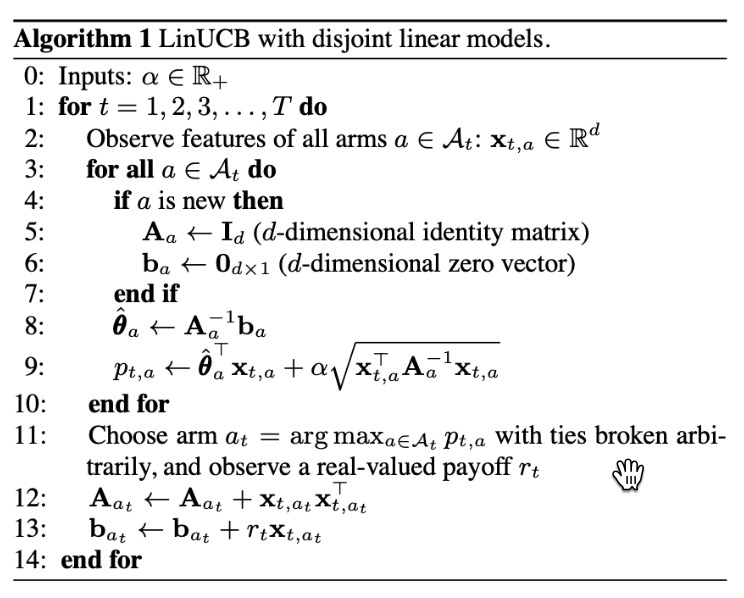# Hybrid LinUCB

Hybrid LinUCB考虑了臂之间的共性，因此每个臂的期望修改为：
$E[r_{t,a} | x_{t,a} ] =z^T_{t,a}\beta^* + x^T_{t,a} \theta^*_a$

• $z_{t,a}$表示的是用户/文章的组合特征
• $\beta^*$表示的是所有臂的共享未知参数
• $\theta^*_a$表示的是臂a的私有参数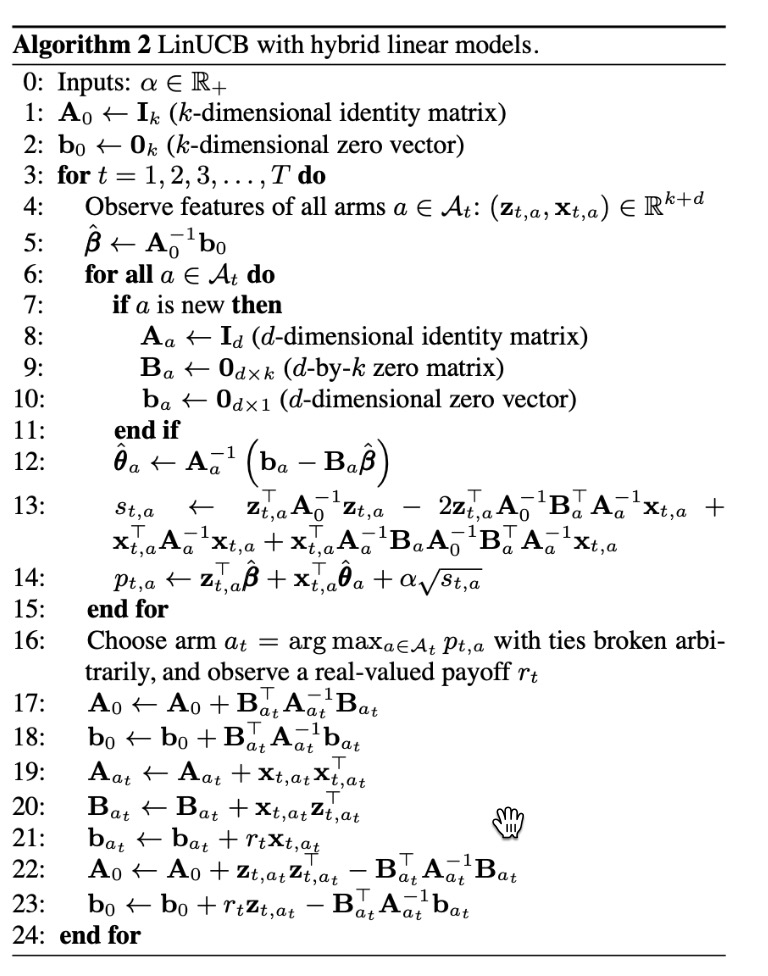# 评估算法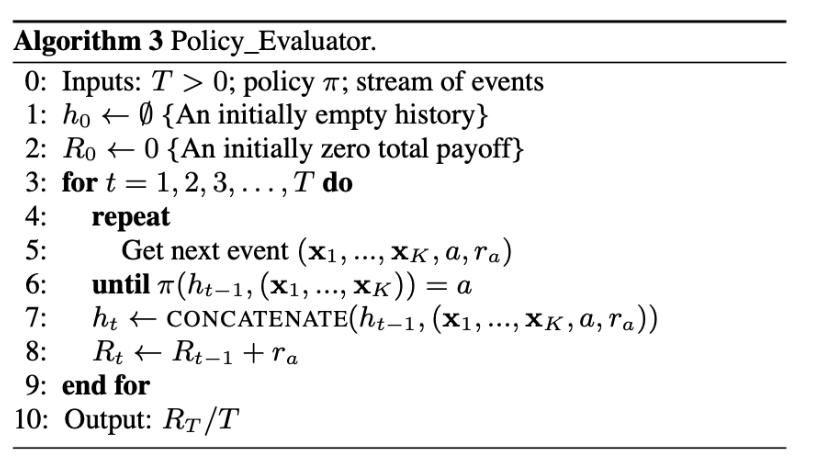• $\pi$为选择的策略，这里即为带上下文的bandit算法
• $h_{t-1}$即为$t-1$时刻的历史行为记录
• $R_T/T$即为最终的实验指标

# 实验说明

## 实验位置## 特征选择

• 用户维度
• 人口统计学信息（性别化为两类，年龄划分为10个等级）
• 地理特征（文中选取的是200个城市地区）
• 行为类别信息（用户的消费历史和属性信息）
• 文章维度：
• 文章URL类别（推断出数十个类别信息）
• 人工编辑的类别信息

# 注意点

• 【实践】在使用Thompson sampling时每个臂的a、b值初始化时要注意，如果差值过大，就会导致经过几次负反馈之后，排在top的臂依旧排在top，因此设计a、b值的时候需要进行特别的设计
• 计算每个臂时的特征为臂a的特征，切勿和臂下的样本特征搞混（如果一个样本是一个臂的话，那么这里的特征就是样本的特征）
• disjoint linucb中的$x_{t,z}$表示的是臂a的特征，$z_{t,a}$表示的是用户/文章的组合特征（臂和文章在某种程度上是一样的概念，比如候选池有100篇文章，那么每篇文章就可以看作是一个臂）
• 读论文时有些论文的实验并不太好做，这时候不仅要学习论文中算法的思路，还要学习论文中实验的设计技巧和评判技巧

# LinUCB 的重点

• LinUCB 不再是上下文无关地，像盲人摸象一样从候选臂中去选择了，而是要考虑上下文因素，比如是用户特征、物品特征和场景特征一起考虑。
• 每一个候选臂针对这些特征各自维护一个参数向量，各自更新，互不干扰。
• 每次选择时用各自的参数去计算期望收益和置信区间，然后按照置信区间上边界最大的输出结果。
• 观察用户的反馈，简单说就是“是否点击”，将观察的结果返回，结合对应的特征，按照刚才给出的公式，去重新计算这个候选臂的参数。
• 当 LinUCB 的特征向量始终取 1，每个候选臂的参数是收益均值的时候，LinUCB 就是 UCB。

2019-12-27 补充

• 当备选池不大的时候，每个备选物品可以作为一个臂，如果备选池大的话可以对备选池的物品进行归类，一个类别作为一个臂
• 不同业务场景下，设置的奖励和惩罚应该是不一样的，比如点击，可以设置为1，购买设置为2，惩罚可以设置为0
• alpha的值通常是拍脑门子定的几个，通过测试比较效果，一般初始值选0.5更加稳妥些

# 场景应用

• 用户维度：性别、年龄、城市、手机品牌等
• 臂维度：曝光点击率、标签类别信息等
• 用户x事物交互维度：用户在该臂下的一些交互维度特征，比如点击、曝光点击率等

• LinUCB不是一个传统的ctr算法，如何与线上服务进行集成？
• 经过onehot或者分桶之后的特征维度会增高，高维的特征必然会引起延迟增大，如何进行线上降维？
• 特征包括了用户访问时携带的特征、臂的离线和实时特征，如何进行特征拼接，进行计算？
• LinUCB和Thomson sampling、UCB相比，参数增多数倍，线上如何进行参数维护和更新？
• 新用户数量往往并不多，在有限的新用户下进行不同留存策略的ABTest，如何保证结果的可靠和有效？
• LinUCB是针对新用户的，那么对于新老用户之间的界定也十分重要，如何界定新老用户或者具体场景下的新老用户？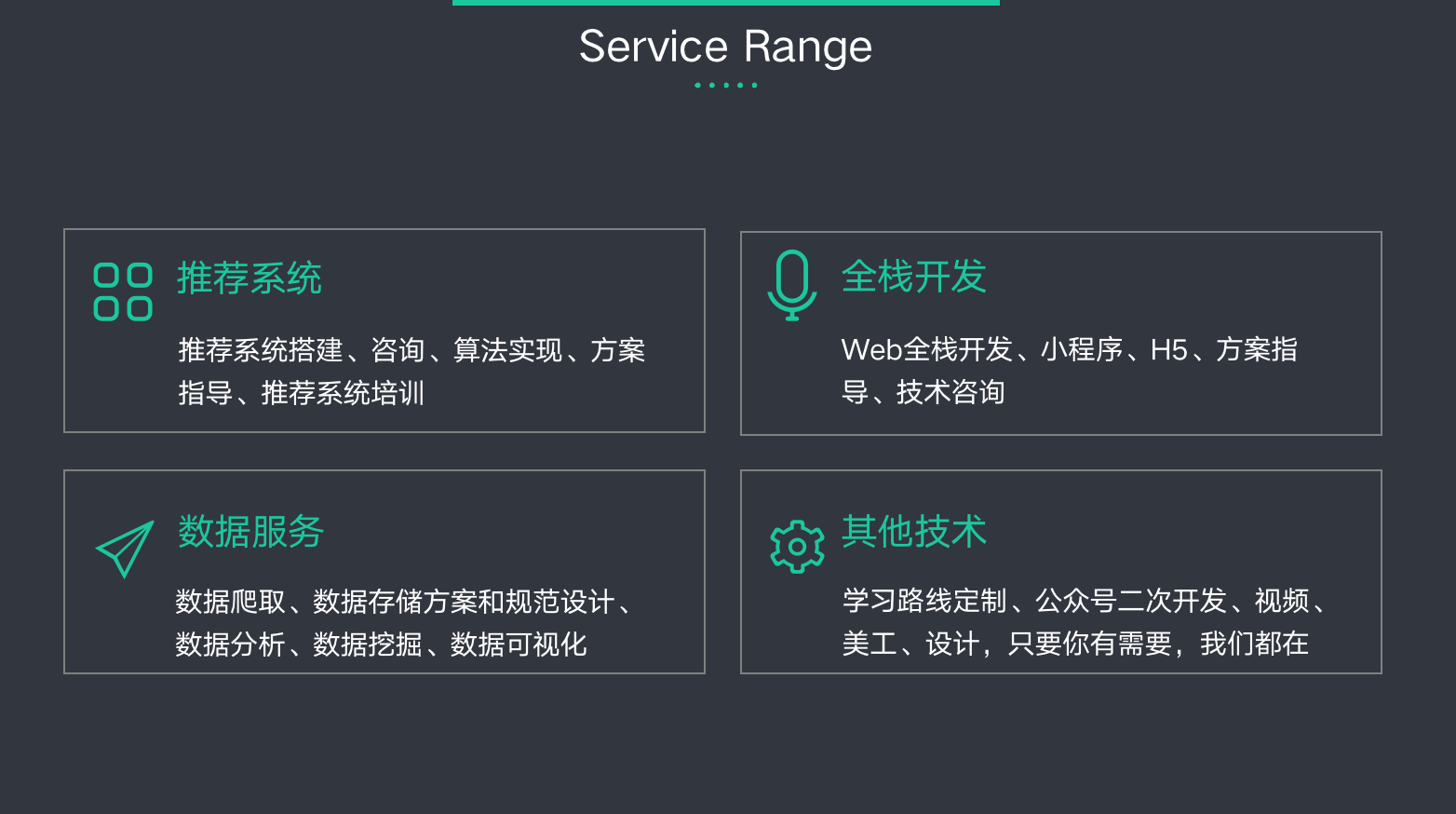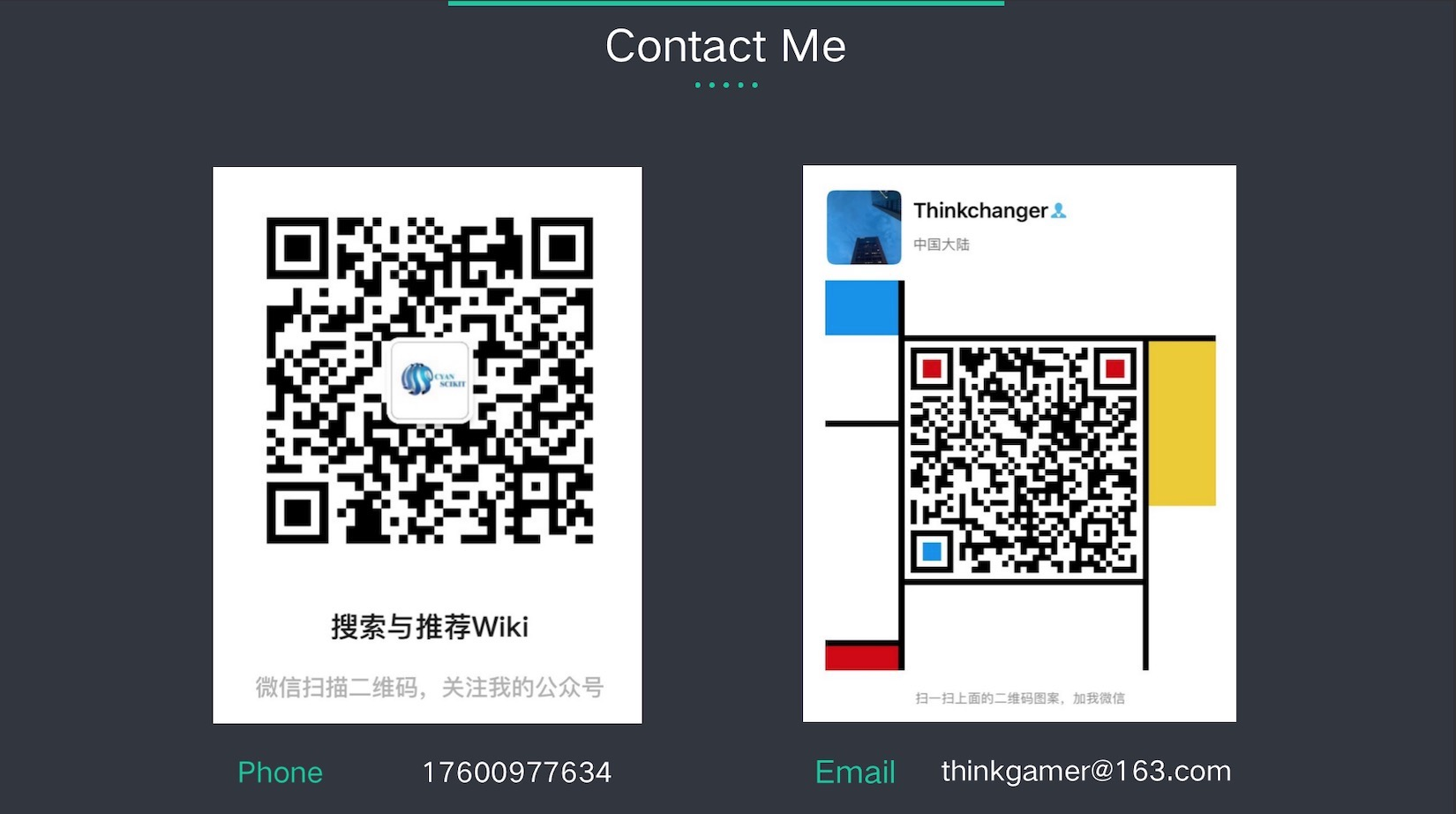©️2019 CSDN 皮肤主题: 大白 设计师: CSDN官方博客